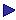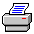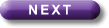Tutorial Contents Tutorial Eight: Relations- Reflexivity - Symmetry -Transitivity - Connectedness - Example of a tableau proof - Eqivalent Relations More Tutorials OneTwoThreeFourFiveSixReflexivity

Rxy is reflexive just if  "xRxx

Examples: "x=y" is reflexive in every domain; "x is the same age as y" is reflexive in the domain of living things, but not, however, in a domain which contains numbers  since, presumably, the number 5 is not the same age as itself, since it isn't any age at all.

So notice that a relation may have a property in one domain which it lacks in others.

Rxy is irreflexive in a domain just if no dot in its graph has a loop.

That is:

Rxy is irreflexive just if "x¬Rxx

Examples: "x is older than y"; "x>y".

Rxy is non-reflexive just if [\$xRxxÙ\$x¬Rxx].

(We could have said: just if [¬"xRxxÙ¬"x¬Rxx]. Remember that "¬"xj" is equivalent to "\$j".)

Examples: "x likes y" (in a suitable domain); "x=y2" (in a domain containing the natural numbers, for instance).

Notice that in the empty domain a relation will be both reflexive and irreflexive: since there will be no dots at all, it follows that there will be no dots with loops (so it is irreflexive) and no dots without loops (so it will be reflexive).

Remember also that in the empty domain anything beginning """ will be true (as long as it contains no names, in which case the formula can't have an interpretation with an empty domain).Print this page